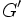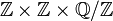# Nilpotent and torsion-free not implies torsion-free abelianization

This article gives the statement, and possibly proof, of the fact that for a group, the subgroup obtained by applying a given subgroup-defining function (i.e., derived subgroup) does not always satisfy a particular subgroup property (i.e., quotient-torsion-freeness-closed subgroup)
View subgroup property satisfactions for subgroup-defining functions$|$ View subgroup property dissatisfactions for subgroup-defining functions

## Statement

It is possible to have a torsion-free nilpotent group$G$ such that the abelianization of$G$ has$p$-torsion for every prime$p$.

In particular, this shows that the derived subgroup in a nilpotent group is not necessarily a quotient-torsion-freeness-closed subgroup.

## Related facts

### Dual fact

Somewhat surprisingly, the dual fact to this is not true. The dual fact, if true, would state that the center of a divisible nilpotent group need not be divisible (and in particular, that the center need not be divisibility-closed in a nilpotent group). This is false. In fact, upper central series members are completely divisibility-closed in nilpotent group.

## Proof

Further information: central product of UT(3,Z) and Q

Let$G$ be the central product of unitriangular matrix group:UT(3,Z) with the group of rational numbers, where the center of the former is identified with a copy of$\mathbb{Z}$ in the latter. Then,

•$G$ is torsion-free.
•$G'$ is isomorphic to$\mathbb{Z}$, and$G/G'$ is isomorphic to$\mathbb{Z} \times \mathbb{Z} \times \mathbb{Q}/\mathbb{Z}$. This has$p$-torsion for all primes$p$.